Mathematics
Easy

Question

# If x and y vary inversely and x = 3 when y =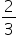, what is the value of y when x = -1 ?

## -2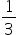-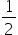-1Hint:

## The correct answer is: -2

### Here, we have to find the value of y given that x and y are in inverse variation.We have, y = k/x=>=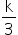=>k = 2.Now, y =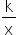=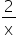=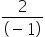= -2Hence, the value of y is -2.The correct option is B.

In inverse variation, xy = k for some constant k.

### Related Questions to study#### With Turito Foundation.#### Get an Expert Advice From Turito.